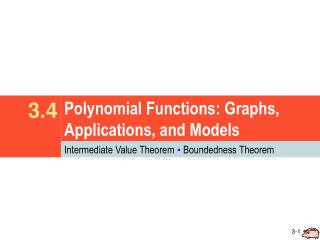Download PresentationPolynomial Functions: Graphs, Applications, and Models

# Polynomial Functions: Graphs, Applications, and Models - PowerPoint PPT Presentation

Polynomial Functions: Graphs, Applications, and Models. 3.4. Intermediate Value Theorem ▪ Boundedness Theorem. Intermediate Value Theorem.I am the owner, or an agent authorized to act on behalf of the owner, of the copyrighted work described.
Download Presentation## Polynomial Functions: Graphs, Applications, and Models

Download Policy: Content on the Website is provided to you AS IS for your information and personal use and may not be sold / licensed / shared on other websites without getting consent from its author.While downloading, if for some reason you are not able to download a presentation, the publisher may have deleted the file from their server.

- - - - - - - - - - - - - - - - - - - - - - - - - - E N D - - - - - - - - - - - - - - - - - - - - - - - - - -
Presentation Transcript
1. Polynomial Functions: Graphs, Applications, and Models 3.4 Intermediate Value Theorem ▪ Boundedness Theorem

2. Intermediate Value Theorem • If f(x) defines a polynomial function with only real coefficients, and if for real numbers a and b, the values of f(a) and f(b) are opposite in sign, then there exists at least one real zero between a and b. • Use synthetic division (twice) to show f(a) and f(b) are opposite in sign. • State this as your conclusion.

3. Locating a Zero Use synthetic division to show that has a real zero between 1 and 2. State the conclusion in the answer. f(1) = 2 > 0 and f(2) = −3 < 0,  by the IVT a real zero between 1 and 2. Use synthetic division to find f(1) and f(2). f(1) = 2 f(2) = –3 "Therefore" “there exists"

4. Locating a Zero f(2) = −1 < 0 and f(3) = 7 > 0,  by the IVT a real zero between 2 and 3. Use synthetic division to show that f(x) = x3 – 2x2 – x + 1 has a real zero between 2 and 3. State the conclusion in the answer. Use synthetic division to find f(2) and f(3). f(2) = –1 f(3) = 7

5. Boundedness Theorem • Let f(x) be a polynomial function of degree n≥ 1 with real coefficients and with a positive leading coefficient. If f(x) is divided synthetically by x – c, and • (a) if c > 0 and all numbers in the bottom row of synthetic division are nonnegative, then f(x) has no zero greater than c; • (b)if c < 0 and the numbers in the bottom row of the synthetic division alternate in sign (with 0 considered positive or negative, as needed), then f(x) has no zero less than c.

6. Using the Boundedness Theorem Show that has no real zero greater than 1. f(x) has real coefficients and the leading coefficient, 1, is positive, so the Boundedness Theorem applies. Divide f(x) synthetically by x – 1. 1 > 0 and all the numbers in the last row are nonnegative, f(x) has no real zero greater than 1.

7. Using the Boundedness Theorem Show that has no real zero less than –2. f(x) has real coefficients and the leading coefficient, 1, is positive, so the Boundedness Theorem applies. Divide f(x) synthetically by x + 2. –2 < 0 and the numbers in the last row alternate in sign,  f(x) has no real zero less than –2.

8. Assignment • Sec. 3.4: pp. 352-353 (47-63 odd) • For 47-55 odd: Use synthetic division twice • For 57-63 odd: Use synthetic division once. • Due Friday, 07 March 2014. • WS Sec. 3.4 (Day 3) • Has all question types that will be on the quiz. • Due Monday, 10 March 2014. • QUIZ: Sec. 3.4 on Friday. • No calculator!!!

9. Sec. 3.4: pp. 352 – 353 (47-63 odd) Due Friday ! ! ! • Use the intermediate value theorem for polynomials to show that each polynomial function has a real zero between the numbers given.

10. Sec. 3.4: pp. 352 – 353 (47-63 odd) Due Friday ! ! ! • Show that the real zeros of each polynomial function satisfy the given conditions.